×#### Thank you for registering.

One of our academic counsellors will contact you within 1 working day.

Click to Chat

1800-1023-196

+91-120-4616500

CART 0

• 0

MY CART (5)

Use Coupon: CART20 and get 20% off on all online Study Material

ITEM
DETAILS
MRP
DISCOUNT
FINAL PRICE
Total Price: Rs.

There are no items in this cart.
Continue Shopping• Complete JEE Main/Advanced Course and Test Series
• OFFERED PRICE: Rs. 15,900
• View Details

```Chapter 8: Quadratic Equations Exercise – 8.7

Question: 1

Find the consecutive numbers whose squares have the same sum of 85.

Solution:

Let the two consecutive two natural numbers be (x) and (x +1) respectively.

Given,

That the sum of their squares is 85.

Then, by hypothesis, we get, = x2 + (x + 1)2 = 85

= x2 + x2 + 2x + 1 = 85

= 2x2 + 2x + 1 - 85 = 0

= 2x2 + 2x + - 84 = 0

= 2(x2 + x + -42) = 0

Now applying factorization method, we get, = x2 + 7x - 6x - 42 = 0

= x(x + 7) - 6(x + 7) = 0

= (x - 6)(x + 7) = 0

Either, x - 6 = 0  therefore, x = 6 x + 7 = 0 therefore x = -7

Hence the consecutive numbers whose sum of squares is 85 are 6 and -7 respectively.

Question: 2

Divide 29 into two parts so that the sum of the squares of the parts is 425.

Solution:

Let the two parts be (x) and (29 - x) respectively.

According to the question, the sum of the two parts is 425.

Then by hypothesis, = x2 + (29 - x)2 = 425

= x2 + x2 + 841 + -58x = 425

= 2x2 - 58x + 841 - 425 = 0

= 2x2 - 58x + 416 = 0

= x2 - 29x + 208 = 0

Now, applying the factorization method = x2 - 13x - 16x + 208 = 0

= x(x - 13) - 16(x - 13) = 0

= (x - 13)(x - 16) = 0

Either x - 13 = 0 therefore x = 13 Or, x - 16 = 0 therefore x = 16

The two parts whose sum of the squares is 425 are 13 and 16 respectively.

Question: 3

Two squares have sides x cm and (x + 4) cm. The sum of their areas is 656 cm2.find the sides of the squares.

Solution:

Given,

The sum of the sides of the squares are = x cm and (x + 4) cm respectively.

The sum of the areas = 656 cm2

We know that, Area of the square = side * side

Area of the square = x(x + 4) cm2

Given that the sum of the areas is 656 cm2

Hence by hypothesis, = x(x + 4) + x(x + 4) = 656

= 2x(x + 4) = 656 = x2 + 4x = 328

Now by applying factorization method, = x2 + 20x - 16x - 328 = 0

= x(x + 20) - 16(x + 20) = 0

= (x + 20)(x –16) = 0

Either x + 20 = 0 therefore x = – 20 Or, x - 16 = 0 therefore x = 16

No negative value is considered as the value of the side of the square can never be negative.

Therefore, the side of the square is 16.

Therefore, x + 4 = 16 + 4 = 20 cm

Hence, the side of the square is 20 cm.

Question: 4

The sum of two numbers is 48 and their product is 432. Find the numbers.

Solution:

Given the sum of two numbers is 48.

Let the two numbers be x and 48 - x also the sum of their product is 432.

According to the question = x(48 - x) = 432

= 48x - x2 = 432

= x2 - 48x + 432 = 0

= x2 - 36x - 12x + 432 = 0

= x(x - 36) - 12(x - 36) = 0

= (x - 36)(x - 12) = 0

Either x - 36 = 0 therefore x = 36 Or, x - 12 = 0 therefore x = 12

The two numbers are 12 and 36 respectively.

Question: 5

If an integer is added to its square, the sum is 90. Find the integer with the help of quadratic equation.

Solution:

Let the integer be x

Given that if an integer is added to its square, the sum is 90

= x + x2 = 90

= x2 + x - 90 = 0

= x2 + 10x - 9x - 90 = 0

= x(x + 10) - 9(x + 10) = 0

= (x + 10)(x - 9) = 0

Either x + 10 = 0

Therefore x = –10 Or, x – 9 = 0

Therefore x = 9

The values of the integer are 9 and -10 respectively.

Question: 6

Find the whole numbers which when decreased by 20 is equal to69 times the reciprocal of the numbers.

Solution:

Let the whole number be x cm

As it is decreased byNow by applying factorization method,

x2 - 23x + 3x - 69 = 0

x(x - 23) + 3(x - 23) = 0

(x - 23)(x + 3) =0

Either, x = 23 Or, x = -3

As the whole numbers are always positive x = – 3 is not considered. The whole number is 23.

Question: 7

Find the consecutive natural numbers whose product is 20

Solution:

Let the two consecutive natural number be x and x + 1 respectively.

Given that the product of natural numbers is 20 = x(x + 1) = 20

= x2 + x - 20 = 0

= x2 + 5x - 4x - 20 = 0

= x(x + 5) - 4(x + 5) = 0

= (x + 5)(x - 4) = 0

Either x + 5 = 0

Therefore x = – 5

Considering the positive value of x. Or, x - 4 = 0

Therefore x = 4

The two consecutive natural numbers are 4 and 5 respectively.

Question: 8

The sum of the squares of two consecutive odd positive integers is 394. Find the two numbers?

Solution:

Let the consecutive odd positive integer are 2x - 1 and 2x + 1 respectively.

Given, that the sum of the squares is 394.

According to the question,

(2x - 1)2 + (2x + 1)2 = 394

4x2 +1 - 4x + 4x2 +1 + 4x = 394

Now cancelling out the equal and opposite terms,

8x2 + 2 = 394

8x2 = 392

x2 = 49

x = 7 and – 7

Since the value of the edge of the square cannot be negative so considering only the positive value.

That is 7 Now,

2x - 1 = 14 -1 = 13

2x + 1 = 14 + 1 = 15

The consecutive odd positive numbers are 13 and 15 respectively.

Question: 9

The sum of two numbers is 8 and 15 times the sum of the reciprocal is also 8. Find the numbers.

Solution:

Let the numbers be x and 8 - x respectively.

Given that the sum of the numbers is 8 and 15 times the sum of their reciprocals.

According to the question,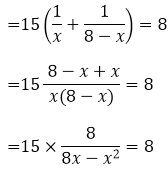= 120 = 8(8x - x2)

= 120 = 64x - 8x2

= 8x2 - 64x + 120 = 0

= 8(x2 - 8x + 15) = 0

= x2 - 8x + 15 = 0

= x2 - 5x - 3x + 15 = 0

= x(x - 5) - 3(x - 5) = 0

= (x - 5)(x - 3) = 0

Either x - 5 = 0 therefore x = 5 Or, x - 3 = 0 therefore x = 3

The two numbers are 5 and 3 respectively.

Question: 10

The sum of a number and its positive square root is 6/25. Find the numbers.

Solution:

Let the number be x

By the hypothesis, we have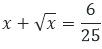Let us assume that x = y2,

we get y + y2 = 6/25

= 25y2 + 25y - 6 = 0

The value of y can be determined by:Where a = 25, b = 25, c = – 6y = 1/5 and y = −11/10 = x = y2 = 15/2 =1/25

The number x is 1/25

Question: 11

There are three consecutive integers such that the square of the first increased by the product of the other two integers gives 154. What are the integers?

Solution:

Let the three consecutive numbers be x, x + 1, x + 2 respectively.

= x2 + (x + 1)(x + 2) = 154

= x2 + x2 + 3x + 2 = 154

= 3x2 + 3x - 152 = 0

The value of x can be obtained by the formula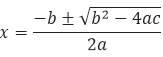Here a = 3, b = 3, c = 152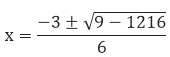x = 8 and x = – 19/2

Considering the value of x If x = 8 x + 1 = 9 x + 2 = 10

The three consecutive numbers are 8, 9, 10 respectively.

Question: 12

The product of two successive integral multiples of 5 is 300. Determine the multiples.

Solution:

Given that the product of two successive integral multiples of 5 is 300

Let the integers be 5x and 5(x+1)

According to the question,

5x[5(x + 1)] = 300

= 25x(x + 1) = 300

= x2 + x = 12

= x2 + x - 12 = 0

= x2 + 4x - 3x - 12 = 0

= x(x + 4) - 3(x + 4) = 0

= (x + 4)(x - 3) = 0

Either x + 4 = 0

Therefore x = -4 Or, x - 3 = 0

Therefore x = 3 x = – 4

5x = – 20

5(x + 1) = -15

x = 3

5x = 15

5(x + 1) = 20

The two successive integral multiples are 15, 20 and -15 and -20 respectively.

Question: 13

The sum of the squares of two numbers is 233 and one of the numbers is 3 less than the other number. Find the numbers.

Solution:

Let the number is x Then the other number is 2x - 3

According to the question: x2 + (2x - 3)2 = 233

= x2 + 4x2 + 9 - 12x = 233

= 5x2 - 12x - 224 = 0

The value of x can be obtained by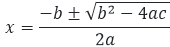Here a = 5, b = – 12, c = – 224x = 8 and x = −28/5

Considering the value of x = 8 2x – 3 = 15 The two numbers are 8 and 15 respectively.

Question: 14

The difference of two number is 4. If the difference of the reciprocal is 421. Find the numbers.

Solution:

Let the two numbers be x and x - 4 respectively.

Given, that the difference of two numbers is 4.

By the given hypothesis we have,= 84 = 4x(x - 4)

= x2 - 4x - 21 = 0

Applying factorization theorem, = x2 - 7x + 3x - 21 = 0

= (x - 7)(x + 3) = 0

Either x - 7 = 0 therefore x = 7 Or, x + 3 = 0 therefore x = -3 Hence the required numbers are – 3 and 7 respectively.

Question: 15

Let us find two natural numbers which differ by 3 and whose squares have the sum 117.

Solution:

Let the numbers be x and x - 3

According to the question x2 + (x - 3)2 = 117

= x2 + x2 + 9 - 6x - 117 = 0

= 2x2 - 6x - 108 = 0

= x2 - 3x - 54 = 0

= x2 - 9x + 6x - 54 = 0

= x(x - 9) + 6(x - 9) = 0

= (x - 9)(x + 6) = 0

Either x - 9 = 0 therefore x = 9 Or, x + 6 = 0 therefore x = – 6

Considering the positive value of x that is 9 x = 9 x - 3 = 6 The two numbers are 6 and 9 respectively.

Question: 16

The sum of the squares of these consecutive natural numbers is 149. Find the numbers.

Solution:

Let the numbers be x, x + 1, and x + 2 respectively.

According to given hypothesis x2 + (x +1 )2 + (x + 2)2 = 149

x2 + x2 + x2 + 1 + 2x + 4 + 4x = 149

3x2 + 6x - 144 = 0

x2 + 2x - 48 = 0

Now applying factorization method, x2  + 8x - 6x - 48 = 0

x(x + 8) - 6(x + 8) = 0

(x + 8)(x - 6) = 0

Either x + 8 = 0 therefore x = – 8 Or, x - 6 = 0 therefore x = 6

Considering only the positive value of x that is 6 and discarding the negative value. x = 6 x + 1 = 7 x + 2 = 8

The three consecutive numbers are 6, 7, and 8 respectively.

Question: 17

Sum of two numbers is 16. The sum of their reciprocal is 1/3.find the numbers.

Solution:

Given that the sum of the two natural numbers is 16 Let the two natural numbers be x and 16-x respectively
According to the question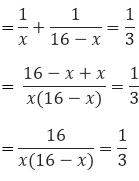= 16x - x2 = 48

= -16x + x2 + 48 = 0

= +x2 - 16x + 48 = 0

= +x2 - 12x - 4x + 48 = 0

= x(x - 12) - 4(x - 12) = 0

= (x - 12)(x - 4) = 0

Either x - 12 = 0 therefore x = 12 Or, x - 4 = 0 therefore x = 4

The two numbers are 4 and 12 respectively.

Question: 18

Determine the two consecutive multiples of 3 whose product is 270.

Solution:

Let the consecutive multiples of 3 are 3x and 3x + 3

According to the question 3x(3x + 3) = 270

= x(3x + 3) = 90

= 3x2 + 3x = 90

= 3x2 + 3x - 90 = 0

= x2 + x - 30 = 0

= x2 + 6x - 5x - 30 = 0

= x(x + 6) - 5(x + 6) = 0

= (x + 6)(x - 5) = 0

Either x + 6 = 0 therefore x = – 6 Or, x - 5 = 0 therefore x = 5

Considering the positive value of x

x = 5 , 3x = 15,  3x + 3 = 18

The two consecutive multiples of 3 are 15 and 18 respectively.

Question: 19

The sum of a number and its reciprocal is 17/4. Find the numbers.

Solution:

Let the number be x According to the question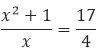= 4(x2+1) = 17x

= 4x2 + 4 - 17x = 0

= 4x2 + 4 - 16x - x = 0

= 4x(x - 4) - 1(x - 4) = 0

= (4x - 1)(x - 4) = 0

Either x - 4 = 0 therefore x = 4 Or, 4x - 1 = 0

therefore x = 1/4 The value of x is 4

Question: 20

A two digit is such that the products of its digits is 8when 18 is subtracted from the number, the digits interchange their places. Find the number?

Solution:

Let the digits be x and x - 2 respectively.

The product of the digits is 8 According to the question x(x - 2) = 8

= x2 - 2x - 8 = 0

= x2 - 4x + 2x - 8 = 0

= x(x - 4) + 2(x - 4) = 0

Either x - 4 = 0 therefore x = 4 Or, x + 2 = 0 therefore x = -2

Considering the positive value of x = 4 x - 2 = 2

The two digit number is 42.

Question: 21

A two digit number is such that the product of the digits is 12, when 36 is added to the number, the digits interchange their places .find the number.

Solution:

Let the tens digit be x Then, the unit digit = 12/x

Therefore the number = 10x + 12/x

And, the number obtained by interchanging the digits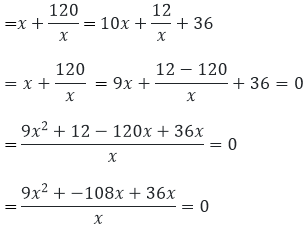= 9(x2 + 4x - 12) = 0

= (x2 + 4x - 12) = 0

= x2 + 6x - 2x - 12 = 0

= x(x + 6) - 2(x + 6) = 0

= (x - 2)(x + 6) = 0

Either x - 2 = 0 therefore x = 2 Or, x + 6 = 0 therefore x = – 6

Since a digit can never be negative. So x = 2

The number is 26.

Question: 22

A two digit number is such that the product of the digits is 16 when 54 is subtracted from the number, the digits are interchanged. Find the number.

Solution:

Let the two digits be: Tens digit be x Units digit be 16/x

Numbers = 10x + 16/x …. (i)

Number obtained by interchanging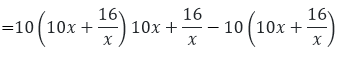= 54 = 10x2 + 16 - 160 + x2 = 54

= 9x2 - 54x - 144 = 0

= x2 - 6x - 16 = 0

= x2 - 8x + 2x - 16 = 0

= x(x - 8) + 2(x - 8) = 0

=(x - 8)(x + 2) = 0

Either x - 8 = 0 therefore x = 8 Or, x + 2 = 0 therefore x = – 2

A digit can never be negative so x = 8

Hence by putting the value of x in the above equation (i) the number is 82.

Question: 23

Two numbers differ by 3 and their product is 504. Find the numbers.

Solution:

Let the numbers be x and x – 3 respectively.

According to the question = x(x - 3) = 504

= x2 - 3x - 504 = 0

= x2 - 24x + 21x - 504 = 0

= x(x - 24) + 21 (x - 24) = 0

= (x - 24)(x + 21) = 0

Either x - 24 = 0 therefore x = 24 Or, x + 21 = 0, therefore x = -21 x = 24 and x = -21 x - 3 = 21 and x – 3 = – 24

The two numbers are 21 and 24 and – 21 and – 24 respectively.

Question: 24

Two numbers differ by 4 and their product is 192. Find the numbers.

Solution:

Let the two numbers be x and x-4 respectively

Given that the product of the numbers is 192

According to the question = x(x - 4) = 192 = x2 - 4x - 192 = 0

= x2 - 16x + 12x - 192 = 0

= x(x - 16) + 12(x - 16) = 0

= (x - 16) (x + 12) = 0

Either x - 16 = 0 therefore x = 16 Or, x + 12 = 0 therefore x = -12

Considering only the positive value of x

x = 16

x - 4 = 12

The two numbers are 12 and 16 respectively.

Question: 25

A two digit number is 4 times the sum of its digits and twice the product of its digits. Find the numbers.

Solution:

Let the digit in the tens and the units place be x and y respectively.

Then it is represented by 10x + y

According to the question,

10x + y = 4(sum of the digits) and 2xy

10x + y = 4(x + y) and 10x + y = 2xy

10x + y = 4x + 4y and 10x + y = 2xy

6x – 3y = 0 and 10x + y – 2xy = 0

y = 2x and 10x + 2x – 2x(2x) = 0

12x = 4x2

4x(x- 3) = 0

Either 4x = 0 therefore x = 0 Or, x –3 = 0 therefore x = 3 We have y = 2x When x = 3, y= 6

Question: 26

The sum of the squares of two positive integers is 208. If the square of the large number is 18 times the smaller. Find the numbers.

Solution:

Let the smaller number be x Then, square of the large number be = 18x

Also, square of the smaller number be = x2

It is given that the sum of the square of the integer is 208.

Therefore,

= x2 + 18x = 208

= x2 + 18x - 208 = 0

Applying factorization theorem,

= x2 + 26x - 8x - 208 = 0

= x(x + 26) - 8(x + 26) = 0

= (x + 26)(x - 8) = 0

Either x + 26 = 0 therefore x = -26 Or, x - 8 = 0 therefore x = 8

Considering the positive number, therefore x = 8.

Square of the largest number = 18x = 18 × 8 = 144

Largest Number = √144 = 12

Hence the numbers are 8 and 12 respectively.

Question: 27

The sum of two numbers is 18. The sum of their reciprocal is 1/4 .find the numbers.

Solution:

Let the numbers be x and (18 – x) respectively.

According to the given hypothesis,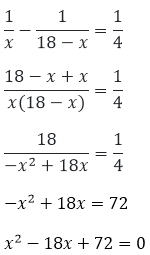Applying factorization theorem, we get,

= x2 - 6x - 12x + 72 = 0

= x(x - 6) - 12(x - 6) = 0

= (x - 6)(x - 12) = 0

Either, x = 6 Or, x = 12

The two numbers are 6 and 12 respectively.

Question: 28

The sum of two numbers a and b is 15 and the sum of their reciprocals 1/a and 1/b is 3/10. Find the numbers a and b.

Solution:

Let us assume a number x such that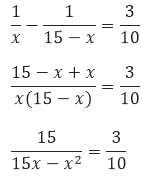= 3x2 - 45x + 150 = 0

= x2 - 15

x + 50 = 0

Applying factorization theorem, = x2- 10x - 5x + 50 = 0

= x(x - 10) - 5(x - 10) = 0

= (x - 10)(x - 5) = 0

Either, x - 10 = 0 therefore x = 10 Or, x - 5 = 0 therefore x = 5

Case (i) If x = a, a = 5 and b = 15 – x , b= 10

Case (ii) If x = 15 - a = 15 - 10 = 5, x = a = 10, b = 15 - 10 = 5

Hence when a = 5, b = 10 a = 10, b = 5

Question: 29

The sum of two numbers is 9. The sum of their reciprocal is 1/2. Find the numbers.

Solution:

Given that the sum of the two numbers is 9
Let the two number be x and 9 - x respectively
According to the question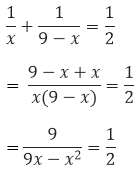= 9x - x2 = 18

= x2 - 9x + 18 = 0

= x2 - 6x - 3x + 18 = 0

= x(x - 6) - 3(x - 6) = 0

= (x - 6)(x - 3) = 0

Either x - 6 = 0 therefore x = 6 Or x - 3 = 0 therefore x = 3

The two numbers are 3 and 6 respectively.

Question: 30

Three consecutive positive integers are such that the sum of the squares of the first and the product of the other two is 46. Find the integers.

Solution

Let the consecutive positive integers be x, x + 1, x + 2 respectively

According to the question X2 + (x + 1)(x + 2) = 46

= x2 + x2 + 3x + 2 = 46

= 2x2 + 3x + 2 = 46

= 2x2 + 3x + 2 - 46 = 0

= 2x2 - 8x + 11x + – 44 = 0

= 2x(x - 4) + 11(x - 4) = 0

= (x - 4)(2x + 11) = 0

Either x - 4 = 0 therefore x = 4 Or, 2x + 11 = 0 therefore x = −11/2

Considering the positive value of x that is x = 4

The three consecutive numbers are 4, 5 and 6 respectively

Question: 31

The difference of squares of two numbers is 88. If the large number is 5 less than the twice of the smaller, then find the two numbers.

Solution:

Let the smaller number be x and larger number is 2x - 5

It is given that the difference of the squares of the number is 88

According to the question (2x - 5)2 - x2 = 88

= 4x2 + 25 - 20x - x2 = 88

= 3x2 - 20x - 63 = 0

= 3x2 - 27x + 7x - 63 = 0

= 3x(x - 9) + 7(x - 9) = 0

= (x - 9)(3x + 7) = 0

Either x - 9 = 0 therefore x = 9 Or, 3x + 7 = 0 therefore x = −7/3

Since a digit can never be negative so x = 9

Hence the number is 2x - 5 = 13

The required numbers are 9 and 13 respectively

Question: 32

The difference of squares of two numbers is180. The square of the smaller number is 8 times the larger number. Find the two numbers

Solution:

Let the number be x

According to the question X2 - 8x = 180

X2 - 8x - 180 = 0

= X2 + 10x - 18x - 180 = 0

= x(x + 10) - 18(x - 10) = 0

= (x - 18)(x + 10) = 0

Either x - 18 = 0 therefore x = 18 Or, x + 10 = 0 therefore x = -10 Case (i) X = 18 8x = 144

Larger number = √144 = 12

Case (ii) X = -10

Square of the larger number 8x= -80

Here in this case no perfect square exist

Hence the numbers are 18 and 12 respectively.

```### Course Features

• 728 Video Lectures
• Revision Notes
• Previous Year Papers
• Mind Map
• Study Planner
• NCERT Solutions
• Discussion Forum
• Test paper with Video Solution